# Beyond Mapping III

Topic 10: Analyzing Map Similarity and Zoning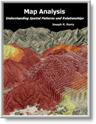Map Analysis book with

companion CD-ROM for

hands-on exercises

describes several techniques for comparing discrete maps
describes several techniques for comparing map surfaces
discusses the underlying concepts in assessing correlation among maps
describes a procedure for deriving a spatial prediction model

Note: The processing and figures discussed in this topic were derived using MapCalcTM software.  See www.innovativegis.com to download a free MapCalc Learner version with tutorial materials for classroom and self-learning map analysis concepts and procedures.

______________________________

Compare Maps by the Numbers

(GeoWorld, September 1999, pg. 24-25)

I bet you've seen and heard it a thousand times¾a speaker waves a laser pointer at a couple of maps and says something like "see how similar the patterns are."  But what determines similarity?  A few similarly shaped gobs appearing in the same general area?  Do all of the globs have to kind of align?  Do display factors, such as color selection, number of classes and thematic break-points, affect perceived similarity?  What about the areas that misalign?

Like abstract art, the patterns formed by map features are subject to subjectivity.  The power of suggestion plays an important role and the old adage that "I wouldn't have seen it, if I hadn't believed it" often holds.  Suggestion can influence map interpretation.  But in visual map comparison, it dominates.

So how can we objectively assess map similarity?  Like most things GIS, it's in the numbers.  Consider the three maps on the right side of figure 1.  Are they similar?  Is the top map more similar to the middle one, than the map on the bottom?  If so, how much more similar?

Actually the three maps were derived from the same map surface.  Map1 identifies low response (lightest tone) as values below 65, medium as values in the range 65 through 70, and high as values over 70 (darkest tone).  Map2 extends the mid-range to 62.5 through 72.5, while Map3 increases it even further to 60 through 75.  In reality all three maps are supposed to be tracking the same spatial variable.  But the categorized renderings appear radically different; or are they surprisingly similar?  What's your visceral vote?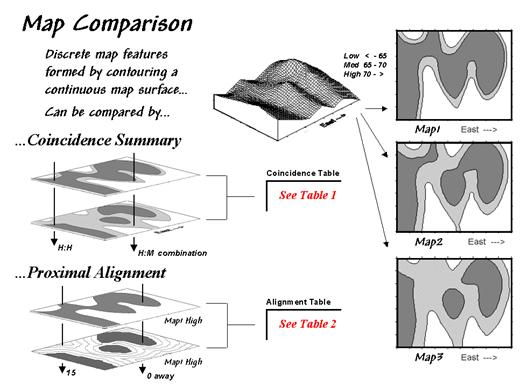Figure 1.  Coincidence Summary and Proximal Alignment can be used to assess the similarity between maps.

One way to find out for certain is to overlay the two maps and note where the classifications are the same and where they are different.  At one extreme, the maps could perfectly coincide with the same conditions everywhere (identical).  At the other extreme, the conditions might be different everywhere.  Somewhere in between these extremes, the high and low areas could be swapped and the pattern inverted¾similar but opposite.

Coincidence summary generates a cross-tabular listing of the intersection of the two maps.  In vector analysis the two maps can be "topologically" overlaid and the areas of the resulting son/daughter polygons aggregated by their joint condition.  Another approach establishes a systematic or random set of points that uses a "point in polygon" overlay to identify/summarize the conditions on both maps.

Raster analysis uses a similar approach but simply counts the number of cells within each category combination as depicted by the arrows in the figure.  In the example, a 39 by 50 grid was used to generate a comprehensive sample set of 1,950 locations (cells).  Table 1 reports the coincidence summaries for the top map with the middle and bottom maps.

The highlighted counts along the diagonals of the table report the number of cells having the same classification on both maps.  The off-diagonal counts indicate disagreement.  The percent values in parentheses report relative coincidence.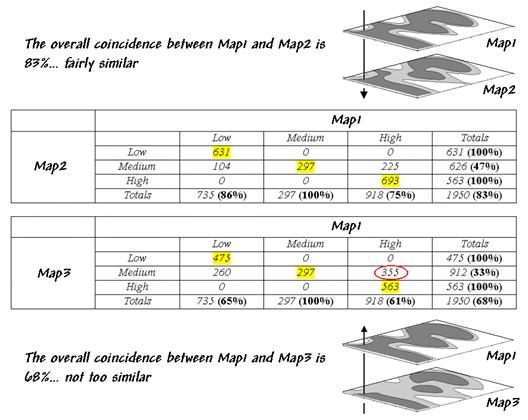Table 1.  Coincidence Summary.

For example, the 100% in the first row indicates that all of "Low" areas on Map2 coincide with "Low" areas on Map1.  The 86% in the first column, however, notes that not all of the "Low" areas on Map1 are classified the same as the same as those on Map2.  The lower portion of the table summarizes the coincidence between Map1 and Map2.

So what do all the numbers mean
¾in user-speak?  First, the "overall" coincidence percentage in the lower right corner gives you a general idea of how well the maps match; 83% is fairly similar, while 68% is not too similar.  Inspection of the individual percentages gives you a handle on which categories are, or are not lining-up.  A perfect match would have 100% for each category; a complete mismatch would have 0%.

But simple coincidence summary just tells you whether things are the same or different.  One extension considers the thematic difference.  It notes the disparity in mismatched categories with a "Low/High" combination considered even less similar than a "Low/Medium" match.

Another procedure investigates the spatial difference, as shown in table 2.  The technique, termed proximal alignment, isolates one of the map categories (the dark-toned areas on Map3 in this case) then generates its proximity map.  The proximity values are "masked" for the corresponding feature on the other map (enlarged dark-toned area on Map1 High).  The highlighted area on the Masked Proximity Map identifies the locations of the greatest misalignment.  Their relative occurrence is summarized in the lower portion of the tabular listing.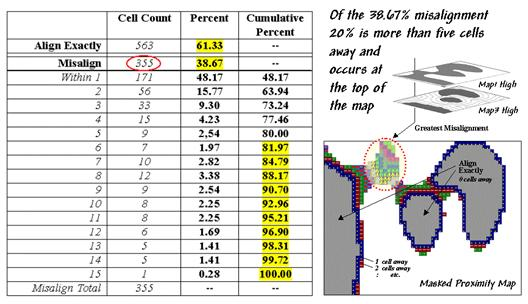Table 2.  Proximal Alignment.

So what does all this tell us¾in user-speak?  First, note that twenty percent of the total mismatches occurs more than five cells away from the nearest corresponding feature, thereby indicating fairly poor overall alignment.  A simple measure of misalignment can be calculated by weight-averaging the proximity information¾1*171 + 2*56 +   / 15 = 3.28.  Perfect alignment would result in 0, with larger values indicating progressively more misalignment.  Considering the dimensionality of the grid (39 x 50), a generalized proximal alignment index can be calculated ¾3.28 / (39*50)**.5 = .074.

So what's the bottom line?  If you’re a GIS software provider, you should include a "big button" for comparing maps with your next release.  If you’re a GIS user, you should report coincidence percentages and alignment indices before passing comparative judgment.  If you’re a laser-waving presenter, your days are numbered.

Use Statistics to Compare Map Surfaces

(GeoWorld, October 1999, pg. 24-25)

While the human brain is good at lot of things, objective and detailed comparison among maps isn't one of them.  Quantitative techniques provide a foothold for map comparison beyond waving a laser-pointer over a couple of maps and boldly stating "see how similar (or dissimilar) the patterns are."

Last month's column identified a couple of techniques for comparing maps composed of discrete map "objects"
¾Coincidence Summary and Proximal Alignment.  Comparing map "surfaces" involves similar approaches, but employs different techniques taking advantage of the more robust nature of continuous data.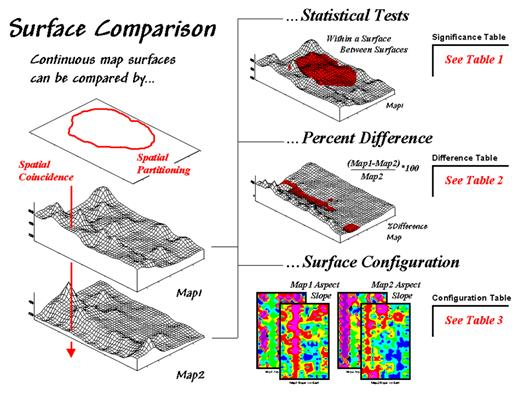Figure 1.  Map surfaces can be compared by statistically testing for significant differences in data sets, differences in spatial coincidence, or surface configuration alignment.

Consider the two map surfaces shown on the left side of figure 1.  Are they similar, or different?  Where are they more similar or different?  Where's the greatest difference?  How would you know?  In visual comparison, your eye looks back-and-forth between the two surfaces attempting to compare the relative "heights" at corresponding locations on the map.  After about ten of these eye-flickers your patience wears thin and you form a hedged opinion¾"not too similar."

In the computer, the relative "heights" are stored as individual map values (in this case, 1380 numbers in an analysis grid of 46 rows by 30 columns).  One thought might be to use statistical tests to analyze whether the data sets are "significantly different."

Since map surfaces are just a bunch of spatially registered numbers, the sets of data can be compared by spatial coincidence (comparing corresponding values on two maps) and spatial partitioning (dividing the mapped data into subsets, then comparing the partitioned areas within one surface or between two surfaces).

In this approach, GIS is used to "package" the data and pass it to standard statistical procedures for analysis of differences within and between data groups.  The packaging can be done in a variety of ways including systematic/random sampling, specified administration/management zones, or inferred spatial groupings.  For example, a scientist could randomly sample values for terrain in two watersheds then test to see if their means are significantly different.  Or a power company could investigate for significant change in household energy consumption for a neighborhood between two billing periods.

Or a farmer could test whether there is a significant difference in the topsoil versus substrata potassium levels for a portion of a field.  Actually, this is the case depicted in figure 1 (Map1 = topsoil; Map2 = substrata potassium) and summarized in table 1.  The dark red area on the surface locates the partitioned area in the field.  The "box-and-whisker" plots in the table identify the mean (dot), +/- standard deviation (shaded box) and min/max values (whiskers) for each of the four data sets (Maps1&2 and in&out of the Partition). Generally speaking, if the boxes tend to align there isn't much of a difference between data groups (e.g., Map2_inP and Map2_outP surfaces).  If they don't align (e.g., Map1_inP and Map2_inP surfaces), there is a significant difference.  The plots provide useful pictures of data distributions and allow you to eyeball the overall differences among a set of map surfaces.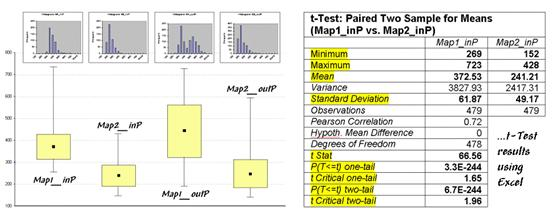Table 1.  Statistical Tests.

The most commonly used statistical method for evaluating the differences in the means of two data groups is the t-test.  The right side of table 1 shows the results of a t-test comparing the partitioned data between Map1_inP and Map2_inP (the first and second box-whisker plots).

While a full explanation of statistical tests is beyond the scope of this discussion, it's relative safe to say the larger the "t stat" value the greater the difference between two data groups.  The values for the "one- and two-tail" tests at the bottom of the table suggest that "the means of the two groups appear distinct and there is little chance that there is no difference between the groups."
As with all things statistical, there are a lot of preconditions that need to be met before a t-test is appropriate
¾the data must be independent and normally distributed.  The problem is that these conditions rarely hold for mapped data.   While the t-test example might serve as a reasonable instance of "blindly applying" non-spatial, statistical tests to mapped data, it suggests this approach is a bit shaky as it seldom provides a reliable test like it does in traditional, non-spatial statistics (see author's notes).

In addition to data condition problems, statistical tests ignore the explicit spatial context of the data.  Comparison using percent difference, on the other hand, capitalizes on this additional information in map surfaces.  Table 2 shows a categorized rendering and tabular summary of the percent difference between the Map1 and Map2 surfaces at each grid location.  Note that the average difference is fairly large (76% +/- 49%), while two identical surfaces would compute to 0% average difference with +/- 0% standard deviation.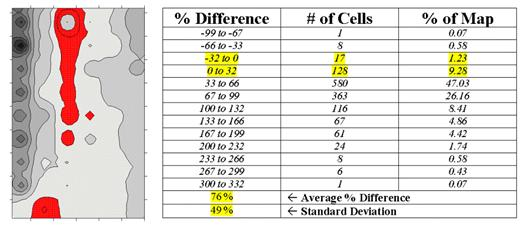Table 2.  Percent Difference.

The dark red areas along the center crease of the map correspond to the highlighted rows in the table identifying areas within +/- 33 percent difference (moderate).  That conjures up the "thirds rule of thumb" for comparing map surfaces¾if two-thirds of the map area is within one-third (33 percent) difference, the surfaces are fairly similar; if less than one-third of the area is within one-third difference, the surfaces are fairly different¾generally speaking that is.  In this case only about 11% of the area meets the criteria so the surfaces are "considerably" different.

Another approach termed surface configuration, focuses on the differences in the localized trends between two map surfaces instead of the individual values.  Like you, the computer can "see" the bumps in the surfaces, but it does it with a couple of derived maps.  A slope map indicates the relative steepness while an aspect map denotes the orientation of locations along the surface.  You see a big bump; it sees an area with large slope values at several aspects.  You see a ridge; it sees an area with large slope values at a single aspect.

So how does the computer see differences in the "lumpy-bumpy" configurations of two map surfaces?  Per usual, it involves map-ematical analysis, but in this case some fairly ugly trigonometry is employed (see equations at end of chapter).  Conceptually speaking, the immediate neighborhood around each grid location identifies a small plane with steepness and orientation defined by the slope and aspect maps.  The mathematician simply solves for the normalized difference in slope and aspect angles between the two planes (see author's notes).

For the rest of us, it makes sense that locations with flat/vertical differences in inclination (Slope_Diff = 90o) and diametrically opposed orientations (Aspect_Diff = 180o) are as different as different can get.  Zero differences for both, on the other hand, are as similar as things can get (exactly the same slope and aspect).  All other slope/aspect differences fall some where in between on a scale of 0-100.

The two superimposed maps at the left side of table 3 show the normalized differences in the slope and aspect angles (dark red being very different).  The map of the overall differences in surface configuration (Sur_Fig) is the average of the two maps.  Note that over half of the map area is classified as low difference (0-20) suggesting that the "lumpy-bumpy" areas align fairly well overall.  The greatest differences in surface configuration appear in the northwest portion.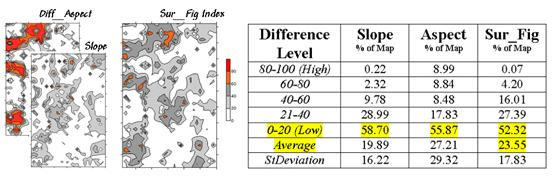Table 3.  Surface Configuration.

Does all this analysis square with your visual inspection of the Map1 and Map2 surfaces in figure 1?  Sort of big differences in the relative values (surface height comparison summarized by percent difference analysis) with smaller differences in surface shape (bumpiness comparison summarized by surface configuration analysis).  Or am I leading the "visually malleable" with quantitative analysis that lacks the comfort, artistry and subjective interpretation of laser-waving map comparison?
_______________________
Author's Notes: An extended discussion by William Huber of Quantitative Decisions on the validity of statistical tests and an Excel workbook containing the equations and computations leading to the t-test, percent difference and surface configuration analyses are available online at the "Column Supplements" page at http://www.innovativegis.com/basis

Use Scatterplots to Understand Map Correlation

(GeoWorld, November 1999, pg. 26-27)

A continuing theme of the Beyond Mapping columns has been that "GIS maps are numbers first, pictures later."  Recent discussions have gone so far as to suggest that there are several ways to quantitatively compare spatial information¾Coincident Summary and Proximal Alignment for discrete maps (see "Comparing Maps," GeoWorld, September, 1999, page ??) and Statistical Tests, Percent Difference and Surface Configuration for continuous map surfaces (see "Comparing Map Surfaces," GeoWorld, October, 1999, page ??).  That brings us to the next big "bungy-jump" in map analysis involving correlation and predictive modeling.

In traditional statistics there is a wealth of procedures for investigating correlation, or "the relationship between variables."  The most basic of these is the scatterplot that provides a graphical peek at the joint responses of paired data.  It can be thought of as an extension of the histogram used to characterize the data distribution for a single variable.

For example, the x- and y-axes in figure 1 summarize the data described last month.  Recall that Map1 identifies the spatial distribution of potassium in the topsoil of a farmer's field, while Map2 tracks the concentrations in the root zone.  Admittedly, this example is “a bit dirty" but keep in mind that a wide array of mapped data from resource managers to market forecasters can be used.

The histograms and descriptive statistics along the axes show the individual data distributions for the partitioned area (dark red "glob" draped on the map surfaces).  It appears the topsoil concentrations in Map1 are generally higher (note the positioning of the histogram peaks; compare the means) and a bit more variable (note the spread of the histograms; compare the standard deviations).  But what about the "joint response" considering both variables at the same time?  Do higher concentrations in the root zone tend to occur with higher concentrations in the topsoil?  Or do they occur with lower concentrations?  Or is there no discernable relationship at all?

These questions involve the concept of correlation that tracks the extent that two variables are proportional to each other.  At one end of the scale, termed positive correlation, the variables act in unison and as values of one variable increase, the values for the other make similar increases.  The other ends, termed negative correlation, the variables are mirrored with increasing values for one matched by decreases in the other.  Both cases indicate a strong relationship between the variables just one is harmonious (positive) while the other is opposite (negative).  In between the two lies no correlation without a discernable pattern between the changes in one variable and the other.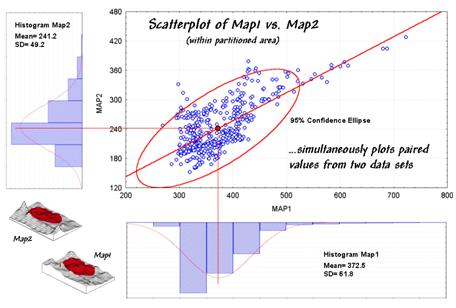Figure 1.  A scatterplot shows the relation between two variables by plotting the paired responses.

Now turn your attention to the scatterplot in figure 1.  Each of the data points (small blue circle) represents one of the 479 grid locations within the partitioned area.  The general pattern of the points provides insight into the joint relationship.  If there is an upward linear trend in the data (like in the figure) positive correlation is indicated.  If the points spread out in a downward fashion there's a negative correlation.  If they seem to form a circular pattern or align parallel to either of the axes, a lack of correlation is noted.

Now let's apply some common sense and observations about a scatterplot.  First the "strength" of a correlation can be interpreted by 1) the degree of alignment of the points with an upward (or downward) line and 2) how dispersed the points are around the line.  In the example, there appears to be fairly strong positive correlation (tightly clustered points along an upward line), particularly if you include the scattering of points along the right side of the diagonal.

But should you include them?  Or are they simply "outliers" (abnormal, infrequent observations) that bias your view of the overall linear trend?  Accounting for outliers is more art than science, but most approaches focus on the dispersion in the vicinity of the joint mean (i.e., statistical "balance point" of the data cloud).  The joint mean in figure 30.3 is at the intersection of the lines extended from the Map1 and Map2 averages.  Now concentrate on the bulk of points in this area and reassess the alignment and dispersion.  Doesn't appear as strong, right?

A quantitative approach to identifying outliers involves a confidence ellipse.  It is conceptually similar to standard deviation as it identifies "typical" paired responses.  In the figure, a 95% confidence ellipse is drawn indicating the area in the plot that accounts for the vast majority of the data.  Points outside the ellipse are candidates for exclusion (25 of 479 in this case) in hopes of concentrating on the overall trend in the data.  The orientation of the ellipse helps you visualize the linear alignment and its thickness helps you visualize the dispersion (pretty good on both counts).

In addition to assessing alignment, dispersion and outliers you should look for a couple of other conditions in a scatterplot
¾distinct groups and nonlinear trends.  Distinct group bias can result in a high correlation that is entirely due to the arrangement of separate data "clouds" and not the true relationships between the variables within each data group.  Nonlinear trends tend to show low "linear" correlation but actually exhibit strong curvilinear relationships (i.e., tightly clustered about a bending line).  Neither of these biases is apparent in the example data.

Now concentrate on the linkage between the scatterplot and the map surfaces.  The analysis grid structures the linkage and enables you to "walk" between the maps and the plot.  If you click on a point in the scatterplot its corresponding cell location on both surfaces are highlighted.  If you click on a location on one of the maps its scatter plot point is highlighted.

That's set the stage for interactive data analysis.  One might click on all of the outlier points and see if they are scattered or grouped.  If they tend to form groups there is a good chance a geographic explanation exists
¾possibly explained by another data layer.

Another investigative procedure is to delineate sets of points on the scatterplot that appear to form "fuzzy globs."  The globs indicate similar characteristics (data pattern) while the map plays out their spatial pattern.  In a sense, manually delineating data globs is analogous to the high-tech, quantitative procedure termed data clustering (see author's notes).  In fact quantitative expression of the scatterplot's correlation information forms the basis for predictive modeling…but that's next month's story.
_______________________
Author's Notes: An extended discussion of data grouping and a online version of "Identifying Data Patterns" (GIS World, August 1998) are available online at the "Column Supplements" page at http://www.innovativegis.com/basis

Can Predictable Maps Work for You?

(GeoWorld, December 1999, pg. 24-25)

The last section discussed map correlation as viewed through a scatterplot.  Recall that the orientation of the "data cloud" indicated the nature of the relationship between the values on two map surfaces, while its shape showed the strength of the relationship.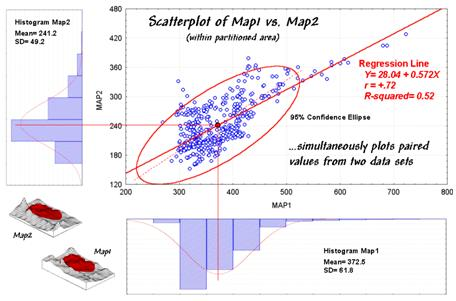Figure 1.  Scatterplot with correlation and regression information identified.

Figure 1 should rekindle the concepts, but note the addition of the information about the regression line.  That brings us to the tough (more interesting?) tuff¾quantitative measures of correlation and predictive modeling.

While a full treatise of the subject awaits your acceptance to graduate school, discussion of some basic measures might be helpful.  The correlation coefficient (denoted as "r") represents the linear relationship between two variables.  It can range from +1.00 (perfect positive correlation) to -1.00 (perfect negative correlation), with a value of 0.00 representing no correlation.  Calculating "r" involves finding the "best-fitting line" that minimizes the sum of the squares of distances from each data point to the line, then summarizing the deviations to a single value.  When the correlation coefficient is squared (referred to as the "R-squared" value), it identifies the "proportion of common variation" and measures the overall strength of the relationship.

The examples in figure 2 match several scatterplots with their R-squared values. The inset on the left shows four scatterplots with increasing correlation (tighter linear alignment in the data clouds).  The middle inset depicts data forming two separate sub-groups (distinct group bias).  In this instance the high R-squared of .81 is misleading.  When the data groups are analyzed separately, the individual R-squared values are much weaker (.00 and .04).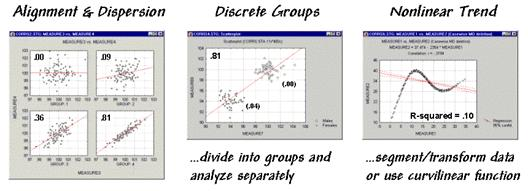Figure 2.  Example scatterplots depicting different data relationships.

The inset on the right is an example of a data pattern that exhibits low linear correlation, but has a strong curvilinear relationship (nonlinear trend).  Unfortunately, dealing with nonlinear patterns is difficult even for the statistically adept.  If the curve is continuously increasing or decreasing, you might try a logarithmic transform; if you can identify the specific function, use it as the line to fit; or, if all else fails, break the curve into segments that are better approximated by a series of straight lines.

Regression analysis extends the concept of correlation by focusing on the best-fitted line (solid red lines in the examples).  The equation of the line generalizes the linear trend in the data.  Also, it serves as a predictive model, while its correlation indicates how good the data fit the model.  In the case of figure 1, the regression equation is
Map2(estimated) = 28.04 + 0.572 * Map1, with an R-squared value of .52.  That means if you measure a potassium level of 500 in the topsoil expect to find about 314 in the root zone (28.04 + 0.572 * 500 = 314.04).  But how good is that guess in the real world?

One way to evaluate the model is to "play-it-back" using the original data.  The left-side of figure 30-6 shows the results for the partitioned area.  As hoped, the predicted surface is very similar to the actual data with an average error of only 2% and over 98% of the area within 33% difference.

Model validation involves testing it on another set of data.  When applied outside the partition the regression model didn't fair as well¾an average error of 19% and only 25% of the area within 33% difference.  The difference surface shows you that the model is pretty good in most places but really blows it along the western edge (big ridge of over estimation) and part of the northern edge (big depression of under estimation).  Maybe those areas should be partitioned and separate prediction models developed for them?  Or, more likely your patience has ebbed.

Figure 3.  Results of applying the predictive model.

A few final concepts should wrap things up.  First, data analysis rarely uses raw data.  As discussed last month "outliers" are identified and eliminated.  In figure 3, the dotted axis through the confidence ellipse suggests a somewhat steeper regression line "better fits" the bulk of the data.  Possibly this equation is a better predictor.

Some data analysts use a "roving window" (e.g., values in a 3x3 adjacent neighborhood) to derive a neighborhood-weighted average for each grid location before deriving a prediction model.  This "smoothing" addresses slight misalignment in the data layers and salt-and-pepper conditions in some data sets.  Another school of thought suggests sampling the data such that the distance between the samples is larger than the spatial autocorrelation as determined by variogram analysis (see "Uncovering the Mysteries of Spatial Autocorrelation," GIS World, June, 1997, page ??).  The sampling interval addresses concern for dependence in the data.

For most of us, however, the bottom-line lies not in debatable statistical theory but in the results.  Regardless of technique, if model validation yields predictions are better than current guesses, then "predictable maps" could work for you.
_______________________

Author's Notes: An Excel workbook extending this discussion to segmented and localized regression is available online at the "Column Supplements" page at http://www.innovativegis.com/basis

Equations for "Comparing Map Surfaces" – Configuration

Using trigonometric relationships to establish differences in surface configuration

Note: Data preparation was completed in MapCalc using an analysis grid configured as 46 rows by 30 rows (1380 map values).

Slope (rate of change) and Aspect (direction of change) maps were derived for both the top and bottom soil potassium maps.

Calculate the "normalized" difference in slope:

Most grid-based GIS packages calculate % slope.

Percent slope can be converted to degrees slope by DEGREES(ARCTAN(%slope/100).

The difference between the two slopes is obtained by ABS(M1_DegSlope - M2_DegSlope).

The difference in slope angles can be normalized between 0 to 100 by

(((Diff_DegSlope - min) * 100) / (max - min))

where min = 0 and max = 90 for degree_slope possible range.

Calculate the "normalized" difference in azimuth:

Most grid-based GIS packages calculate precise aspect in degrees azimuth.

The difference between two azimuths can be calculated in degrees by